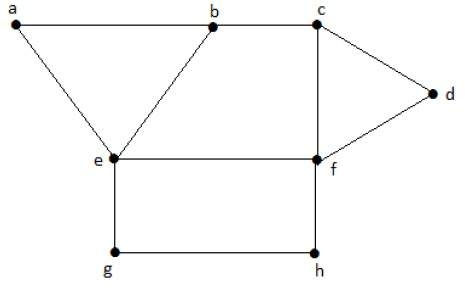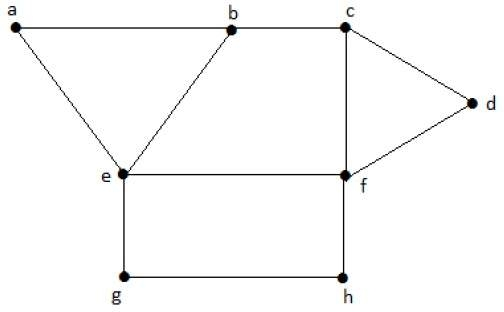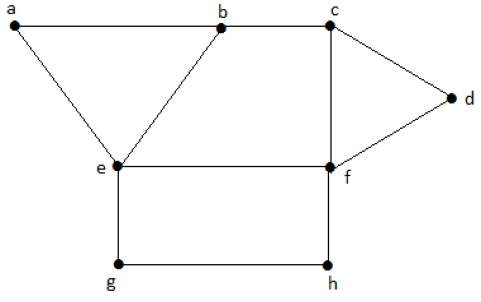# Independent Vertex Set

Independent sets are represented in sets, in which

• there should not be any edges adjacent to each other. There should not be any common vertex between any two edges.

• there should not be any vertices adjacent to each other. There should not be any common edge between any two vertices.

## Independent Vertex Set

Let 'G' = (V, E) be a graph. A subset of 'V' is called an independent set of 'G' if no two vertices in 'S' are adjacent.

### ExampleConsider the following subsets from the above graphs −

S1 = {e}
S2 = {e, f}
S3 = {a, g, c}
S4 = {e, d}

Clearly, S1 is not an independent vertex set, because for getting an independent vertex set, there should be at least two vertices in the form a graph. But here it is not that case. The subsets S2, S3, and S4 are the independent vertex sets because there is no vertex that is adjacent to anyone vertex from the subsets.

## Maximal Independent Vertex Set

Let 'G' be a graph, then an independent vertex set of 'G' is said to be maximal if no other vertex of 'G' can be added to 'S'.

### ExampleConsider the following subsets from the above graphs.

S1 = {e}
S2 = {e, f}
S3 = {a, g, c}
S4 = {e, d}

S2 and S3 are maximal independent vertex sets of 'G'. In S1 and S4, we can add other vertices; but in S2 and S3, we cannot add any other vertex

## Maximum Independent Vertex Set

A maximal independent vertex set of 'G' with a maximum number of vertices is called the maximum independent vertex set.

### ExampleConsider the following subsets from the above graph −

S1 = {e}
S2 = {e, f}
S3 = {a, g, c}
S4 = {e, d}

Only S3 is the maximum independent vertex set, as it covers the highest number of vertices. The number of vertices in a maximum independent vertex set of 'G' is called the independent vertex number of G (β2).

### Example

For the complete graph Kn,
Vertex covering number = α2 = n-1
Vertex independent number = β2 = 1
You have α2 + β2 = n
In a complete graph, each vertex is adjacent to its remaining (n − 1) vertices. Therefore, a maximum independent set of Kn contains only one vertex.
Therefore, β2=1
and α2=|v| − β2 = n-1

Note − For any graph 'G' = (V, E)

• α2 + β2 = |v|

• If 'S' is an independent vertex set of 'G', then (V – S) is a vertex cover of G.